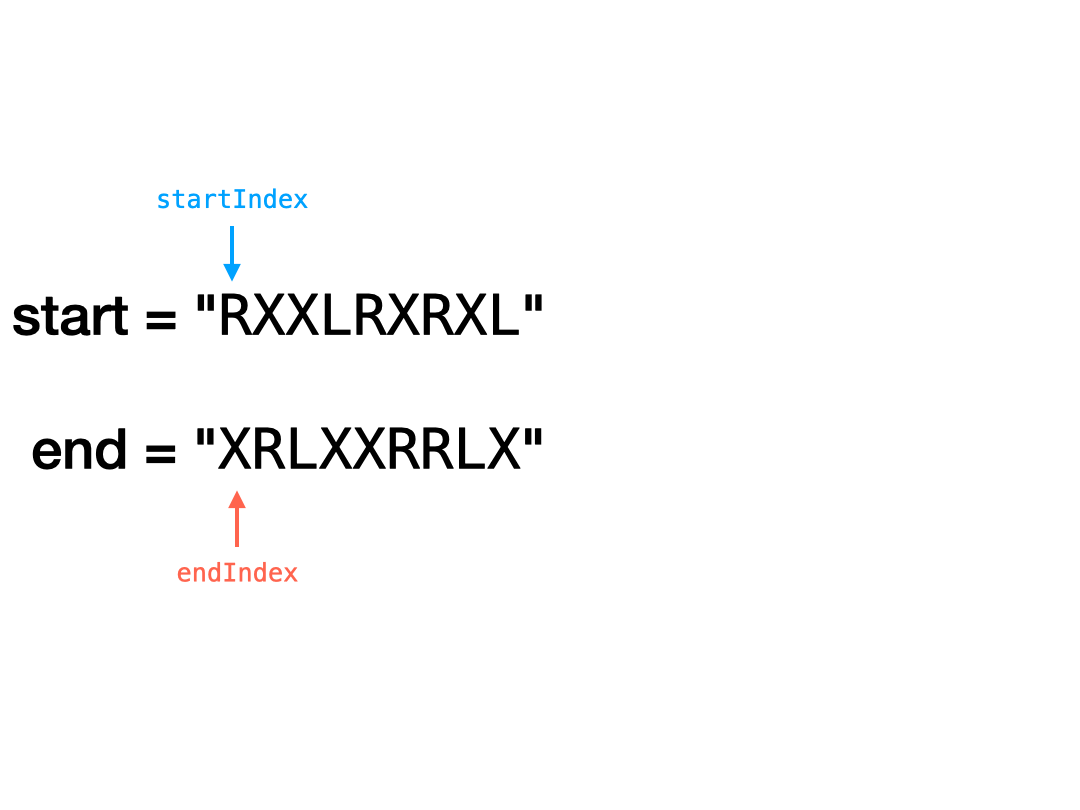# LeetCode 777. Swap Adjacent in LR String Solution

In a string composed of 'L', 'R', and 'X' characters, like "RXXLRXRXL", a move consists of either replacing one occurrence of "XL" with "LX", or replacing one occurrence of "RX" with "XR". Given the starting string start and the ending string end, return True if and only if there exists a sequence of moves to transform one string to the other.

Example 1:

Input: start = "RXXLRXRXL", end = "XRLXXRRLX"
Output: true
Explanation: We can transform start to end following these steps: RXXLRXRXL -> XRXLRXRXL -> XRLXRXRXL -> XRLXXRRXL -> XRLXXRRLX

Example 2:

Input: start = "X", end = "L"
Output: false

## Solution

The first observation we can make is that the two moves can be described as the following: shift L to the left and shift R to the right. Since L and R cannot be swapped with each other, the relative order of L and R letters will never change.

Let's label L and R as valid letters.

Our first condition for a transformation from start to end is that both start and end must have the same number of valid letters. In addition, the first valid letter in start must match the first valid letter in end, the second valid letter in start must match the second valid letter in end, and so on until the last.

We can also observe that for a transformation to exist, the $i^{th}$ valid letter in start must be able to move to the position of the $i^{th}$ valid letter in end. We'll denote startIndex as the index of the $i^{th}$ valid letter in start and endIndex as the index of the $i^{th}$ valid letter in end. There are two cases to consider:

• The valid letter is L. Since L can only move left, a transformation exists when startIndex >= endIndex.
• The valid letter is R. Since R can only move right, a transformation exists when startIndex <= endIndex.

We can implement this using the idea of Two Pointers to keep track of startIndex and endIndex for every valid letter.### Time Complexity

Let's denote $N$ as the length of both strings start and end.

Since we use Two Pointers to iterate through both strings once, our time complexity is $O(N)$.

Time Complexity: $O(N)$

### Space Complexity

Space Complexity: $O(1)$

## C++ Solution

1class Solution {
2   public:
3    bool canTransform(string start, string end) {
4        int n = start.size();
5        int startIndex = 0;
6        int endIndex = 0;
7        while (startIndex < n || endIndex < n) {
8            while (startIndex < n &&
9                   start[startIndex] == 'X') {  // find next valid letter in start
10                startIndex++;
11            }
12            while (endIndex < n &&
13                   end[endIndex] == 'X') {  // find next valid letter in end
14                endIndex++;
15            }
16            if (startIndex == n && endIndex == n) {  // both reached the end
17                return true;
18            }
19            if (startIndex == n || endIndex == n) {  // different number of valid letters
20                return false;
21            }
22            if (start[startIndex] != end[endIndex]) {  // different valid letter
23                return false;
24            }
25            if (start[startIndex] == 'R' && startIndex > endIndex) {  // wrong direction
26                return false;
27            }
28            if (start[startIndex] == 'L' && startIndex < endIndex) {  // wrong direction
29                return false;
30            }
31            startIndex++;
32            endIndex++;
33        }
34        return true;
35    }
36};

## Java Solution

1class Solution {
2    public boolean canTransform(String start, String end) {
3        int n = start.length();
4        int startIndex = 0;
5        int endIndex = 0;
6        while (startIndex < n || endIndex < n) {
7            while (startIndex < n
8                && start.charAt(startIndex) == 'X') { // find next valid letter in start
9                startIndex++;
10            }
11            while (endIndex < n
12                && end.charAt(endIndex) == 'X') { // find next valid letter in end
13                endIndex++;
14            }
15            if (startIndex == n && endIndex == n) { // both reached the end
16                return true;
17            }
18            if (startIndex == n || endIndex == n) { // different number of valid letters
19                return false;
20            }
21            if (start.charAt(startIndex)
22                != end.charAt(endIndex)) { // different valid letter
23                return false;
24            }
25            if (start.charAt(startIndex) == 'R'
26                && startIndex > endIndex) { // wrong direction
27                return false;
28            }
29            if (start.charAt(startIndex) == 'L'
30                && startIndex < endIndex) { // wrong direction
31                return false;
32            }
33            startIndex++;
34            endIndex++;
35        }
36        return true;
37    }
38}

## Python Solution

1class Solution:
2    def canTransform(self, start: str, end: str) -> bool:
3        n = len(start)
4        startIndex = 0
5        endIndex = 0
6        while startIndex < n or endIndex < n:
7            while (
8                startIndex < n and start[startIndex] == "X"
9            ):  # find next valid letter in start
10                startIndex += 1
11            while (
12                endIndex < n and end[endIndex] == "X"
13            ):  # find next valid letter in end
14                endIndex += 1
15            if startIndex == n and endIndex == n:  # both reached the end
16                return True
17            if startIndex == n or endIndex == n:  # different number of valid letters
18                return False
19            if start[startIndex] != end[endIndex]:  # different valid letter
20                return False
21            if start[startIndex] == "R" and startIndex > endIndex:  # wrong direction
22                return False
23            if start[startIndex] == "L" and startIndex < endIndex:  # wrong direction
24                return False
25            startIndex += 1
26            endIndex += 1
27        return True
28